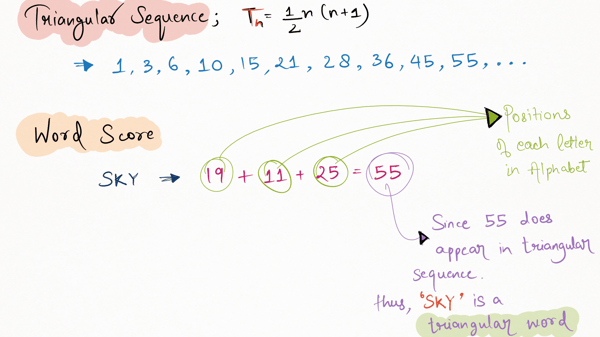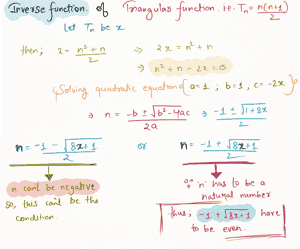Related Tags

project euler
communitycreator

# Project Euler 42: Coded triangle numbers

Armaan Nougai

### Problem statement

The $nth$ term of the triangular sequence is defined as:

 Tn = n*(n+1)/2


Word score is the sum of each of its letter’s positions in the alphabet.

A word is a triangular word if its word score is in a triangular sequence.

Given a text file of nearly 2,000 words, find the number of triangular words in the file.

WORDSQuestion Analysis

### Approach #1

We will simply iterate through each word and keep extending the triangular sequence, such that the word score is less than the last value of the triangular sequence calculated.

While this approach works, it is not efficient.

### Approach #2

In this approach, we do not generate a sequence.

The triangular function is as follows:

Tn = n*(n+1)/2


Where: input is n and output is the $nth$ term in the triangular sequence.

We will inverse this function such that the input will be the word score and the output will be n, and if n is a natural number the word is triangular."""
Coded by - Armaan Nougai
"""
#paste text from the file below

from math import sqrt
Alpha = 'ABCDEFGHIJKLMNOPQRSTUVWXYZ'

word_score = lambda s: sum(map(lambda x: 0 if x not in Alpha else (Alpha.index(x)+1)*s.count(x),set(s) ))
global triangular_sq
triangular_sq = 

def generate(value):
global triangular_sq
term = len(triangular_sq)
while triangular_sq[-1] <= value:
term += 1
triangular_sq += [ triangular_sq[-1]+term ]

with open('__ed_input.txt','r') as FILE:

triangular_words = 0
for y in Data:
ws = word_score(y)
generate(ws)
triangular_words += int(ws in triangular_sq)

print(triangular_words)



Enter the input below to be saved in file __ed_input.txt

"""
Coded by - Armaan Nougai
"""
#paste text from the file below

from math import sqrt
Alpha = 'ABCDEFGHIJKLMNOPQRSTUVWXYZ'

word_score = lambda s: sum(map(lambda x: 0 if x not in Alpha else (Alpha.index(x)+1)*s.count(x),set(s) ))

with open('__ed_input.txt','r') as FILE:
triangular_words = 0
for y in Data:
ws = word_score(y)
approx_n = (sqrt(1+8*ws)-1)
triangular_words += int(approx_n%2==0)

print(triangular_words)

Enter the input below to be saved in file __ed_input.txt

RELATED TAGS

project euler
communitycreator

CONTRIBUTOR

Armaan Nougai
RELATED COURSES

View all Courses

Keep Exploring

Learn in-demand tech skills in half the time